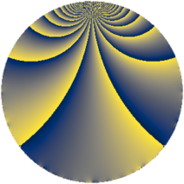# Properties

 Label 91.2.bcLevel $91$ Weight $2$ Character orbit 91.bc Rep. character $\chi_{91}(6,\cdot)$ Character field $\Q(\zeta_{12})$ Dimension $32$ Newform subspaces $1$ Sturm bound $18$ Trace bound $0$

# Learn more

## Defining parameters

 Level: $$N$$ $$=$$ $$91 = 7 \cdot 13$$ Weight: $$k$$ $$=$$ $$2$$ Character orbit: $$[\chi]$$ $$=$$ 91.bc (of order $$12$$ and degree $$4$$) Character conductor: $$\operatorname{cond}(\chi)$$ $$=$$ $$91$$ Character field: $$\Q(\zeta_{12})$$ Newform subspaces: $$1$$ Sturm bound: $$18$$ Trace bound: $$0$$

## Dimensions

The following table gives the dimensions of various subspaces of $$M_{2}(91, [\chi])$$.

Total New Old
Modular forms 48 48 0
Cusp forms 32 32 0
Eisenstein series 16 16 0

## Trace form

 $$32q - 8q^{2} - 12q^{4} - 16q^{8} + 8q^{9} + O(q^{10})$$ $$32q - 8q^{2} - 12q^{4} - 16q^{8} + 8q^{9} - 4q^{11} - 32q^{14} - 8q^{15} + 12q^{16} - 4q^{18} + 16q^{21} + 4q^{22} - 12q^{23} + 24q^{28} + 4q^{29} + 12q^{30} + 4q^{32} - 20q^{35} + 4q^{37} - 36q^{39} - 28q^{42} - 48q^{43} + 24q^{44} + 84q^{46} + 24q^{49} - 44q^{50} + 72q^{53} + 60q^{56} - 92q^{57} - 16q^{58} + 76q^{60} + 48q^{63} + 4q^{65} - 56q^{67} + 56q^{70} + 84q^{71} - 128q^{72} - 24q^{74} + 148q^{78} - 80q^{79} + 28q^{81} - 64q^{84} + 36q^{85} - 48q^{86} - 228q^{88} - 48q^{91} + 24q^{92} + 108q^{93} - 84q^{95} - 32q^{98} - 60q^{99} + O(q^{100})$$

## Decomposition of $$S_{2}^{\mathrm{new}}(91, [\chi])$$ into newform subspaces

Label Dim. $$A$$ Field CM Traces $q$-expansion
$$a_2$$ $$a_3$$ $$a_5$$ $$a_7$$
91.2.bc.a $$32$$ $$0.727$$ None $$-8$$ $$0$$ $$0$$ $$0$$